Home > MC2 > Chapter 11 > Lesson 11.1.2 > Problem11-24

11-24.
1. Simplify each expression. Homework Help ✎

1.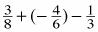2.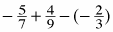3.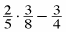4.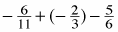5.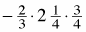6.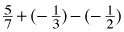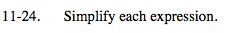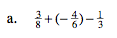Find a common denominator.

$\frac{9}{24} + (- \frac{16}{24}) - \frac{8}{24}$

$\frac{9 - 16 - 8}{24} = \frac{-15}{24}$

Can this fraction be further
reduced?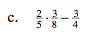Use the order of operations.

$\frac{6}{40} - \frac{3}{4}$

$\frac{6}{40} - \frac{30}{40}$

$\frac{6-30}{40} = \frac{-24}{40} = \frac{-3}{5}$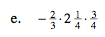Convert the mixed number
into a fraction greater than
one before simplifying.

$- \frac{2}{3} ( \frac{9}{4} ) ( \frac{3}{4} )$

$- \frac{54}{48} = - \frac{9}{8}$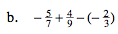See (a).

$\frac{25}{63}$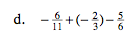See (a).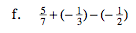See (a).